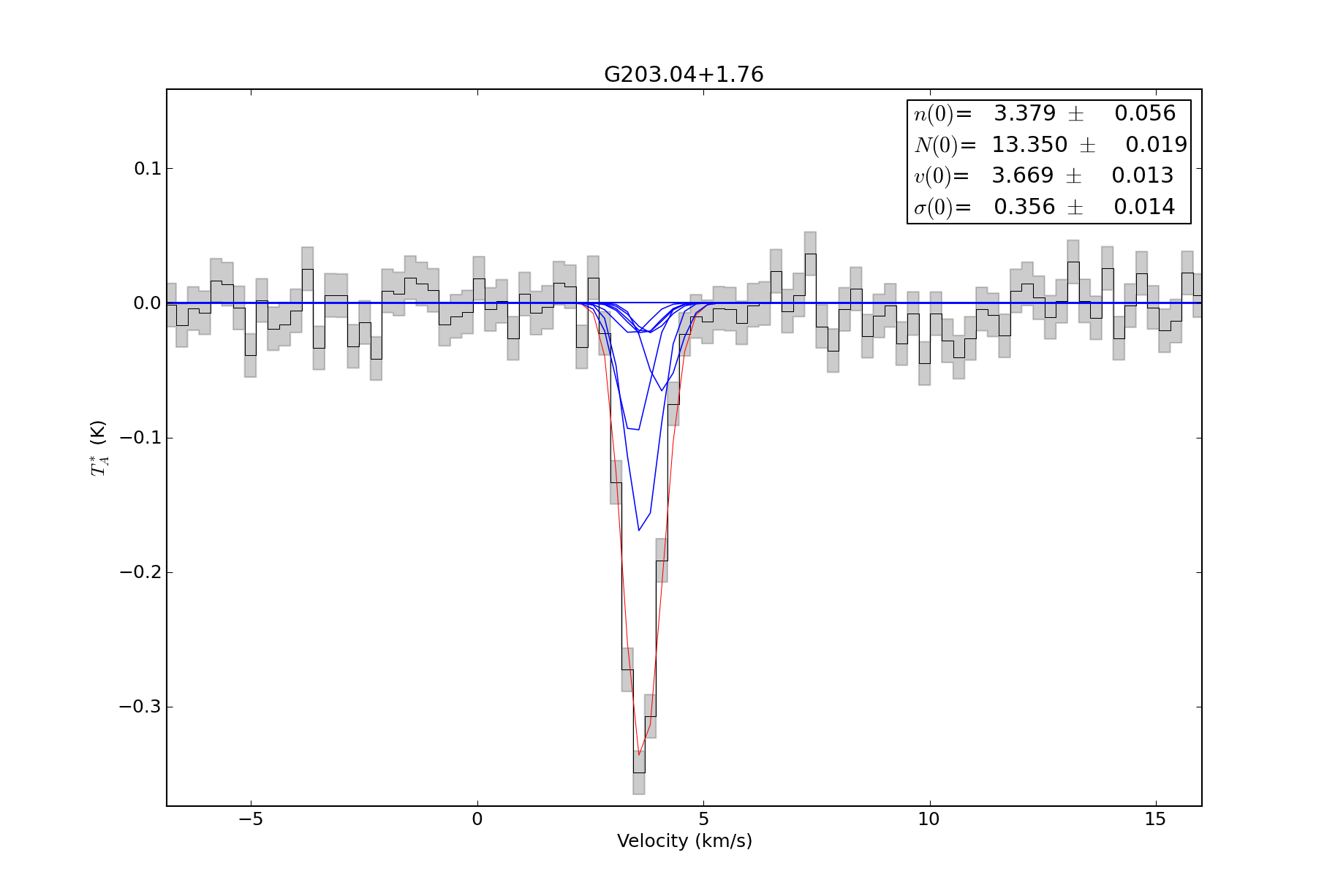Because an LVG model grid is being used as the basis for the fitting in this example, there are fewer free parameters. If you want to create your own model grid, there is a set of tools for creating RADEX model grids (in parallel) at the agpy RADEX page. The model grids used below are available on the pyspeckit bitbucket download page.

```import pyspeckit
import numpy as np
import astropy.io.fits as pyfits
from pyspeckit.spectrum import models

# create the Formaldehyde Radex fitter
# This step cannot be easily generalized: the user needs to read in their own grids

# this deserves a lot of explanation:
# models.formaldehyde.formaldehyde_radex is the MODEL that we are going to fit
# models.model.SpectralModel is a wrapper to deal with parinfo, multiple peaks,
# and annotations
# all of the parameters after the first are passed to the model function
parnames=['density','column','center','width'],
parvalues=[4,12,0,1],
parlimited=[(True,True), (True,True), (False,False), (True,False)],
parlimits=[(1,8), (11,16), (0,0), (0,0)],
parsteps=[0.01,0.01,0,0],
fitunits='Hz',
texgrid=((4,5,texgrid1),(14,15,texgrid2)), # specify the frequency range over which the grid is valid (in GHz)
taugrid=((4,5,taugrid1),(14,15,taugrid2)),
hdr=hdr,
shortvarnames=("n","N","v","\\sigma"), # specify the parameter names (TeX is OK)
grid_vwidth_scale=False,
)

# sphere version:

parnames=['density','column','center','width'],
parvalues=[4,12,0,1],
parlimited=[(True,True), (True,True), (False,False), (True,False)],
parlimits=[(1,8), (11,16), (0,0), (0,0)],
parsteps=[0.01,0.01,0,0],
fitunits='Hz',
texgrid=((4,5,texgrid1),(14,15,texgrid2)),
taugrid=((4,5,taugrid1),(14,15,taugrid2)),
hdr=hdr,
shortvarnames=("n","N","v","\\sigma"),
grid_vwidth_scale=True,
)

sp1 = pyspeckit.Spectrum('G203.04+1.76_h2co.fits',wcstype='D',scale_keyword='ETAMB')
sp2 = pyspeckit.Spectrum('G203.04+1.76_h2co_Tastar.fits',wcstype='V',scale_keyword='ETAMB')

sp1.crop(-50,50)
sp1.smooth(3) # match to GBT resolution
sp2.crop(-50,50)

sp1.xarr.convert_to_unit('GHz')
sp1.specfit() # determine errors
sp1.error = np.ones(sp1.data.shape)*sp1.specfit.residuals.std()
sp1.baseline(excludefit=True)
sp2.xarr.convert_to_unit('GHz')
sp2.specfit() # determine errors
sp2.error = np.ones(sp2.data.shape)*sp2.specfit.residuals.std()
sp2.baseline(excludefit=True)
sp = pyspeckit.Spectra([sp1,sp2])

sp.plotter()

# these are just for pretty plotting:
sp1.specfit.fitter = sp.specfit.fitter
sp1.specfit.modelpars = sp.specfit.modelpars
sp1.specfit.model = np.interp(sp1.xarr,sp.xarr,sp.specfit.model)
sp2.specfit.fitter = sp.specfit.fitter
sp2.specfit.modelpars = sp.specfit.modelpars
sp2.specfit.model = np.interp(sp2.xarr,sp.xarr,sp.specfit.model)

# previously, xarrs were in GHz to match the fitting scheme
sp1.xarr.convert_to_unit('km/s')
sp2.xarr.convert_to_unit('km/s')

sp1.plotter(xmin=-5,xmax=15,errstyle='fill')
sp1.specfit.plot_fit(show_components=True)
sp2.plotter(xmin=-5,xmax=15,errstyle='fill')
sp2.specfit.plot_fit(show_components=True)

sp.plotter(figure=5)

# these are just for pretty plotting:
sp1.specfit.fitter = sp.specfit.fitter
sp1.specfit.modelpars = sp.specfit.modelpars
sp1.specfit.model = np.interp(sp1.xarr.as_unit('GHz'),sp.xarr,sp.specfit.model)
sp2.specfit.fitter = sp.specfit.fitter
sp2.specfit.modelpars = sp.specfit.modelpars
sp2.specfit.model = np.interp(sp2.xarr.as_unit('GHz'),sp.xarr,sp.specfit.model)

sp1.plotter(xmin=-5,xmax=15,errstyle='fill',figure=6)
sp1.specfit.plot_fit(show_components=True)
sp2.plotter(xmin=-5,xmax=15,errstyle='fill',figure=7)
sp2.specfit.plot_fit(show_components=True)
```Both transitions are fit simultaneously using a RADEX model. The input (fitted) parameters are therefore density, column density, width, and velocity.¶